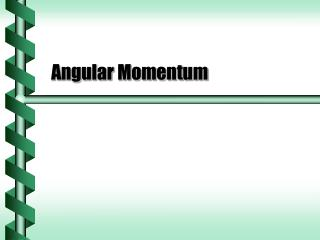DownloadDownload PresentationAngular Momentum

# Angular Momentum

Télécharger la présentation## Angular Momentum

- - - - - - - - - - - - - - - - - - - - - - - - - - - E N D - - - - - - - - - - - - - - - - - - - - - - - - - - -
##### Presentation Transcript

1. Angular Momentum

2. Moment of Momentum • To continue the analysis of rotational motion, we must also extend the idea of momentum. • Define an angular momentum L. • Based on the momentum p = mv • Includes a lever arm • Follows the rules for torque p r

3. Momentum Cross Product • Angular momentum is a vector. • Vector cross product of the lever arm and momentum. • Direction follows the right-hand rule • Magnitude from sine rule L p r

4. Law of Angular Inertia • The time derivative of angular momentum vector is the net torque vector. • By the law of reaction, all internal torques come in canceling pairs. • Add external torques

5. Applying Torque • An external torque changes angular momentum. • dL/dt= t L L+rpsinq w w p

6. A child of 180 N runs at 4.5 m/s and hops on the edge of a merry-go-round with radius 2.0 m. If the motion is perpendicular to the radius, what is angular momentum? The child starts with linear momentum. W = mg, m = W/g = 18 kg. p = mv = 81 kg m/s At right angles, all the linear momentum contributes. L = rp = 160 kg m2/s The torque is due to the friction at contact. Starting the Ride m r

7. Spinning Mass • The moment of inertia is the analog of mass for rotational motion. • The analog for angular momentum would be: w

8. A child of 180 N runs at 3.0 m/s and hops on the edge of a merry-go-round with radius 2.0 m and mass of 160 kg. What is the period of rotation? The moment of inertia and the angular momentum for the child on the ride was found before. I = Id + Ic =390 kg m2 L = rp = 160 kg m2/s The period is related to the angular velocity. L = Iw = I(2p/T) T= 2p I/ L = 15 s Initial Spin m M r

9. Single Axis Rotation • An axis of rotation that is fixed in direction gives a single axis rotation. • Simplest case has the axis through the center of mass • Angular momentum vector is parallel to the angular velocity L w

10. Limitations • There are limitations to the relationship between angular momentum and angular velocity. • Moving axis of rotation • Asymmetric axis of rotation • Angular momentum and angular velocity can have different directions. p L r

11. Angular Momentum Vector • The vector form of the law of rotational action is generalized to use angular momentum vectors. • Correct for all axes • Correct for changes in direction as well as angular velocity next